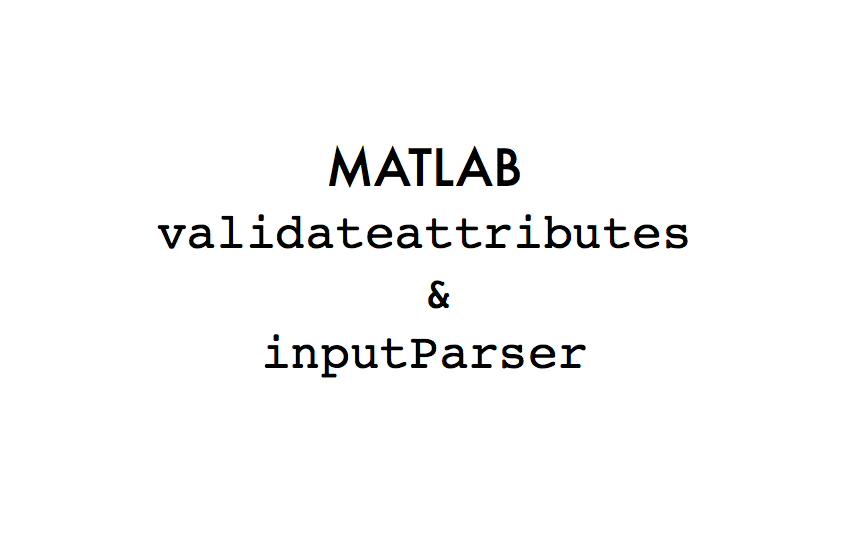• 为什么要对函数的输入进行检查
• validateattributes的基本使用
• validateattributes的额外提示信息
• validateattributes支持的检查类型和属性
• validatestring
• inputParser的基本使用
• inputParser的可选参数和默认参数值设置
• inputParser和validateattributes联合使用
• inputParser的参数名参数值对的设置
• inputParser解析结构体输入
• 引子：为什么需要MATLAB的单元测试系统

## validateattributes的基本使用

``````% 函数一开始检查输入变量的类型和尺寸
function processImg(img)
...
validateattributes(img,{'numeric'},{'size',[500,500]});
... % 函数继续
end
``````

validateattributes的第一个参数img是输入的图像，即要检查的变量；第二个参数是要检查的类型，这里规定img必须是数值类型(numeric);第三个参数对变量要检查的属性，这里的属性是对img规定的尺寸。

validateattributes的最基本调用格式是：

``````validateattributes(A,classes,attributes)
``````

``````%元胞数组中可以放置多个要检查的属性
...
validateattributes(img,{'numeric'},{'size',[500,500],'>=',0,'<=',255});
...
``````

``````% 检查数据的类型是double且单增
...
validateattributes(xgrid,{'double'},{'increasing'})
...
``````

validateattributes最少需要三个参数，如果我们只需要检查变量的类型，则第三个参数可以用空的元胞数组来代替。比如写一个阶乘的函数，其输入必须是无符号的整数，除此之外不做之外的其他检查，可以这样写：

``````% 第三个属性参数为空
...
validateattributes(iA,{'uint8'},{});
``````

``````% MyClass
classdef MyClass
properties
myprop
end
end
``````

``````% 要求变量obj是MyClass类的对象且非空
...
validateattributes(obj,{'MyClass'},{nonempty});
...
``````

## validateattributes的额外提示信息

``````% 一个简化的计算面积的函数
function A = getArea(width,height)
A = width*height;
end
``````

``````function A = getArea(width,height)
validateattributes(width,{'numeric'},{'positive'});
validateattributes(height,{'numeric'},{'positive'});
A = width*height;
end
``````

``````% 命令行测试函数功能
>> getArea(10,22)
ans =
220

>> getArea(10,0)                   % 如预期捕捉到了错误
Error using getArea (line 3)
Expected input to be positive.

>> getArea(0,22)
Error using getArea (line 2)      % 两个错误信息除了行号,都是一样的
Expected input to be positive.
``````

``````% validateattributes支持额外的诊断信息
function A = getArea(width,height)
validateattributes(width, {'numeric'},{'positive'},'getArea','width' ,1);
validateattributes(height,{'numeric'} {'positive'},'getArea','height',2);
A = width*height;                                   %参数4   参数5  参数6
end
``````

``````>> getArea(10,0)
Error using getArea
Expected input number 2, height, to be positive.   清楚的说明getArea函数的
Error in getArea (line 3)                          第2个参数不符合规定
validateattributes(height,{'numeric'},{'positive'},'getArea','height',2);

>> getArea(0,22)
Error using getArea
Expected input number 1, width, to be positive.
Error in getArea (line 2)
validateattributes(width,{'numeric'},{'positive'},'getArea','width',1);
``````

``````% 一共5种调用方式
validateattributes(A,classes,attributes)
validateattributes(A,classes,attributes,argIndex)
validateattributes(A,classes,attributes,funcName)
validateattributes(A,classes,attributes,funcName,varName)
validateattributes(A,classes,attributes,funcName,varName,argIndex)
``````

## validateattributes支持的检查类型和属性

validateattributes可以检查的数据类型：'single','double','int8','int16','int32','int64','uint8','uint16','uint32','uint64','logical','char','struct','cell','function handle','numeric','class name'.

validateattributes可以检查的数据维度属性如下：

validateattributes支持检查的数据的大小范围属性如下：

validateattributes还支持检查的数据其它属性如下：

## validatestring

``````% validatestring基本用法
...
str = validatestring(concentrationUnit,{'ppm','ppb'});
...
``````

``````% command line
>> concentrationUnit= 'ppm';
>> str = validatestring(concentrationUnit,{'ppm','ppb'});
str =
ppm   % concentrationUnit匹配了ppm
``````

``````% command line
>> concentrationUnit= 'pp';
>> str = validatestring(concentrationUnit,{'ppm','ppb'});
Error
Expected input to match one of these strings:
'ppm', 'ppb'
The input, pp, matched more than one valid string.
``````

``````% 输入是全名
>> colorValue = 'green';
>> str = validatestring(colorValue,       {'red','green','blue','cyan','yellow','magenta'})
str =
green
``````

``````% 输入的名字是Inexact Name
>> colorValue = 'G';
>> str = validatestring(colorValue,{'red','green','blue','cyan','yellow','magenta'})
str =
green     % G 匹配了green
``````

``````% 匹配必须是独一无二的
>> in = 'color';
>> str = validatestring(in,{'ColorMap','ColorSpace'})
Expected input to match one of these strings:
'ColorMap', 'ColorSpace'   %color两个都可以匹配
The input, color, matched more than one valid string.
``````

## inputParser的基本使用

``````% getArea的基本形式
function a = getArea(wd,ht)
a = wd*ht;
end
``````

``````% getArea版本1
function a = getArea(wd,ht)

p = inputParser;

p.parse(wd,ht);

a = p.Results.width*p.Results.height;  % 从Results处取结果
end
``````

``````% 命令行验证
>> getArea(10,22)
ans =
220

>> getArea(10)          % 如预期报错 调用少一个参数
Error using getArea
Not enough input arguments.

>> getArea('10',22)     % 如预期报错 参数width类型错误
Error using getArea (line 8)
The value of 'width' is invalid. It must satisfy the function:  isnumeric.
``````

1. 首先第3行声明一个inputParser的对象，等式右边是inputParser的类名称，也是该类的构造函数。
3. 第8行把函数的实参wd,ht提供给inputParser对象，并且进行解析，解析的内容将存放在p.Results中。
4. 第10行从p.Results中取出解析的结果，计算面积并返回。

inputParser是一个MATLAB类，其UML类图如下：

Figure.5, inputParser类图

## inputParser的可选参数和默认参数值设置

``````>> getArea(10)
Error using getArea (line 8)
Not enough input arguments.
``````

`````` % getArea版本2
function a = getArea(width,varargin)

p = inputParser;

defaultheight = width;                           %取默认值为输入的width

p.parse(width,varargin{:});

a = p.Results.width*p.Results.height;
end
``````

1. 第1行中的参数被分成了两个部分，第一个输入width和其余的部分，其余部分的参数被包装在了元胞数组中，后面还会看到更多这样的例子。
2. 第7行指定了可选参数的默认值。
3. 第8行给inputParser添加了height作为可选参数

``````% 命令行测试函数功能
>> getArea(10)    % 正确处理的了单个参数的情况
ans =
100

>> getArea(10,22) % 确保仍然可以处理两个参数的情况
ans =
220
``````

## inputParser和validateattributes联合使用

inputParser的主要功能是对多个输入参数的解析，其对每个参数的值的检查可以使用匿名函数，

``````% getArea版本2
function a = getArea(width,varargin)

p = inputParser;
{'nonzero'},'getArea','width',1));

defaultheight = width;
{'nonzero'},'getArea','height',2));

p.parse(width,varargin{:});
% 注意要把varargin元胞中的内容解开提供给parse函数

a = p.Results.width*p.Results.height;
end
``````

`````` % 命令行测试函数功能
>> getArea(10,0)  % 如预期检查出第二个参数的错误，并给出提示
Error using getArea (line 37)
The value of 'height' is invalid. Expected input number 2, height, to be nonzero.

>> getArea(0,22)  % 如预期检查出第一个参数的错误，并给出提示
Error using getArea (line 37)
The value of 'width' is invalid. Expected input number 1, width, to be nonzero.
``````

## inputParser的参数名参数值对的设置

• 一个叫做shape,用来表示形状，可取的值是rectangle,square和paralelogram. 其默认值是rectangle。
• 另一个叫做unit,用来表示输入的单位，可取的值是cm,m,inches，其默认值是inches

``````% getArea版本3
function r = getArea(width,varargin)

p = inputParser;
{'nonzero'}));

defaultheight = width;
{'nonzero'}));

defaultshape = 'rectangle';
@(x)any(validatestring(x,{'square','rectangle','paralelogram'})));

defaultunit = 'inches';
@(x)any(validatestring(x,{'inches','cm','m'})));

p.parse(width,varargin{:});

r.area =  p.Results.width*p.Results.height;
r.shape = p.Results.shape;  %简单起见，shape和unit作为结构体的中的一部分返回
r.units = p.Results.units;
end
``````

``````% 命令行测试函数功能
>> getArea(10,22,'square')   % 只提供shape
ans =

area: 220
units: 'inches'          % units取默认值
shape: 'square'

>> getArea(10,22,'square','cm')
ans =

area: 220
units: 'cm'
shape: 'square'
``````

``````>> getArea(10,22,'cm','square') % 颠倒了第三和第四个参数
Error using getArea
The value of 'shape' is invalid. Expected input to match one of these strings:
'square', 'rectangle', 'paralelogram'
The input, 'cm', did not match any of the valid strings
``````

（2）如要想给第四个参数提供任何值，必须指定第三个参数的值，尽管第三个参数的值有可能是默认值：

``````>> getArea(10,22,'rectangle','inches')
ans =             %^该值等于默认值

area: 220
units: 'inches'
shape: 'rectangle'
``````

MATLAB的许多函数都不需要记住参数的输入顺序，比如plot函数：

``````x = 0:pi/10:pi;
y = sin(x) ;
plot(x,y,'color','g', 'LineWidth',2,'MarkerSize',10);
``````

``````plot(x,y,'LineWidth',2,'MarkerSize',10,'color','g');
``````

``````% getArea版本3:把之前的addOptional都换成addParameter
function a = getArea(width,varargin)

.....
@(x)any(validatestring(x,{'square','rectangle','paralelogram'})));

....
@(x)any(validatestring(x,{'inches','cm','m'})));
....
end
``````

``````% 命令行测试函数功能
>> getArea(10,22,'shape','square','units','m')
ans =            %--name  value  --name   value
area: 220
shape: 'square'
units: 'm'

>> getArea(10,22,'units','m','shape','square')  % 变化了参数的位置
ans =
area: 220
shape: 'square'
units: 'm'

>> getArea(10,22,'units','m')                   % 仅仅提供unit参数
ans =
area: 220
shape: 'rectangle'
units: 'm'
``````

## inputParser解析结构体输入

``````% inputParser也可以用来解析结构体
function runProgram(configStruct)

p = inputParser;

DefaultMaxIter  = 100  ;  % 计算参数的默认值
DefaultTol      = 0.001;
DefaultStepSize = 0.01 ;

@(x)validateattributes(x,{'numeric'},{'>',0,'real'}));
%迭代次数下限
@(x)validateattributes(x,{'numeric'},{'<=',0.01,'real'}));
%收敛上限
@(x)validateattributes(x,{'numeric'},{'<=',0.01,'real'}));
%步长上限
p.parse(configStruct);

.....
end
``````

``````% 命令行测试函数功能
>> configStruct.MaxIter = 10;
>> configStruct.Tol = 0.001;
>> configStruct.StepSize = 0.01;
>> runProgram(configStruct);

>> configStruct.MaxIter = 10;
>> configStruct.Tol = 0.001;
>>runProgram(configStruct);
``````

## 引子：为什么需要MATLAB的单元测试系统

Figure.6, 函数更新开发流程1

Figure.7, 函数更新开发流程2

Figure.8 函数和函数测试共生的模式

MathWorks开发部MATLAB架构C++高级软件工程师。计算物理学博士，研究方向为电子结构计算、密度泛函算法开发；计算机硕士，研究方向为图像处理。2004年，开始使用MATLAB，在科研编程中遇到了开发大型程序难以维护的困难，花了很多时间用于改进程序但效果总不尽如人意。2009年，开始使用MATLAB面向对象编程，发现工程进度被迅速加快，于是萌生了写一本介绍MATLAB 面向对象编程书的念头。2011年，在美国取得博士学位之后入职MathWorks，从理科科研工作者和多年的MATLAB爱好者，成为一名MATLAB语言的设计开发和实现的软件工程师。2016年，作者在MATLAB中文论坛开辟了技术专栏，和大家分享最新的行业应用技术和MATLAB编程理念，旨在推动软件工程中的现代手段在MATLAB科学工程计算项目中的使用，帮助科学家和工程师们更有效地解决复杂的科研问题。《MATLAB面向对象编程:从入门到设计模式(第二版)》 凝结了作者多年的科研和工作经验以及对MATLAB语言的理解，希望能对各种规模的科学工程计算项目的MATLAB使用者有所启发。